# Worked Solutions 6: Implicit Methods

In :
import numpy as np
import matplotlib.pyplot as plt
%matplotlib inline


We will consider the pair of (linear) first-order ordinary differential equations \begin{eqnarray} \frac{dx}{dt} &=& \phantom{-}398 x + 798 y , \ \frac{dy}{dt} &=& -399 x - 799 y , \quad \quad x(0) = 1, \; \, y(0) = 0 , \end{eqnarray} which define the functions $x(t)$ and $y(t)$.

1(a). Exact solution
The equations can be written in matrix form, as $$\frac{d\mathbf{x}}{dt} = \mathbf{A} \mathbf{x}, \quad \text{where} \quad \mathbf{x} = \begin{pmatrix}x(t) \\ y(t)\end{pmatrix} , \quad \mathbf{A} = \begin{pmatrix} 398 & 798 \\ -399 & -799 \end{pmatrix}.$$

The matrix $\mathbf{A}$ has a pair of eigenvectors $\mathbf{e}_i$ and eigenvalues $\lambda_i$ such that $\mathbf{A} \mathbf{e}_i = \lambda \mathbf{e}_i$. In our case, $\lambda_1 = -400, \lambda_2 = -1$ and $\mathbf{e}_1 = \begin{pmatrix} -1 \\ 1 \end{pmatrix}$, $\mathbf{e}_2 = \begin{pmatrix} 2 \\ -1 \end{pmatrix}$. It is straightforward to show that the general solution of a 2D set of linear ODEs can be written in terms of the eigenvectors and eigenvalues as follows: $$\mathbf{x}(t) = A_1 e^{\lambda_1 t} \mathbf{e}_1 + A_2 e^{\lambda_2 t} \mathbf{e}_2 ,$$ where $A_1$, $A_2$ are constants. The initial condition $\mathbf{x}(0) = \begin{pmatrix} 1 \\ 0 \end{pmatrix}$ implies that $A_1 = A_2 = 1$, which gives the exact solution $x(t) = 2 e^{-t} - e^{-400t}$, $y(t) = -e^{-t} + e^{-400t}$.

(Alternatively, you may have substituted the exact solution back into the ODEs to verify that it is a valid solution.)

In :
ts = np.linspace(0,1,200)
xexact = 2*np.exp(-ts) - np.exp(-400*ts)
yexact = -np.exp(-ts) + np.exp(-400*ts)
texact = ts
plt.plot(texact, xexact, 'r-', texact, yexact, 'b-');
# Note the rapid change at the beginning of the interval
plt.title("Exact solution");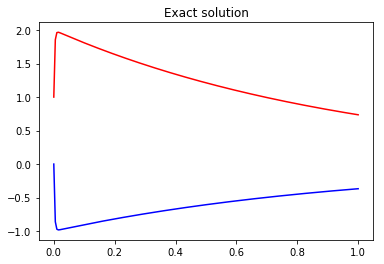In :
def forwardEuler(h=1/100, tstart = 0.0, tend = 1.0):
n = int((tend - tstart) / h)
ts = np.linspace(tstart, tend, n+1)
xs = np.zeros(n+1); ys = np.zeros(n+1);
xs = 1.0; ys = 0.0;  # Set the initial condition here
for k in range(n):
xs[k+1] = xs[k] + h*( 398*xs[k] + 798*ys[k])
ys[k+1] = ys[k] + h*(-399*xs[k] - 799*ys[k])
return ts, xs, ys

# Choosing h = 1/200 leads to a numerical solution with a spurious oscillation, as shown
# Choosing a larger step size leads to exponential growth (instability).
ts,xs,ys = forwardEuler(h=1/200)
plt.xlabel("t");
plt.plot(ts, xs, 'r-', ts, ys, 'b-');
plt.plot(texact, xexact, 'r-', texact, yexact, 'b-');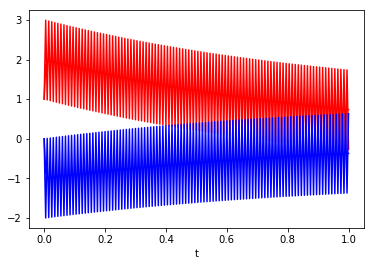In :
def backwardEuler(h=1/100, tstart = 0.0, tend = 1.0):
n = int((tend - tstart) / h)
ts = np.linspace(tstart, tend, n+1)
xs = np.zeros(n+1); ys = np.zeros(n+1);
xs = 1.0; ys = 0.0;  # Set the initial condition here
Delta = 1 + 401*h + 400*h**2
for k in range(n):
xs[k+1] = 1/Delta*((1+799*h)*xs[k] + 798*h*ys[k])
ys[k+1] = 1/Delta*(-399*h*xs[k] + (1-398*h)*ys[k])
return ts, xs, ys

# With the backward Euler method, there is no sign of instability,
# even for large step sizes.
ts,xs,ys = backwardEuler(h=1/200)
plt.plot(ts, xs, 'r-', ts, ys, 'b-');
plt.plot(texact, xexact, 'r-', texact, yexact, 'b-');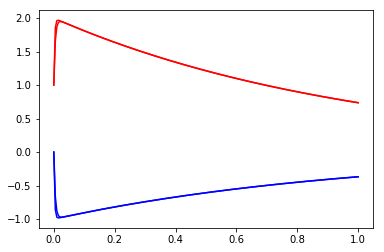In :
# Implicit methods can have better stability properties than explicit methods;
# but they are less straightforward to implement for non-linear ODEs, as a method
# is needed to solve the implicit equations


# 2. Multi-step methods¶

In :
# 2(a)
def f(x,t):
return -x + (1+ np.tanh(t-20.0))*np.sin(t)
def twostep(h=0.02, tmax=60):
tmin = -h
n = int((tmax-tmin)/h)
ts = tmin + h*np.arange(n+1)
xs = np.zeros(n+1)
# we need two initial conditions
# to get started; fortunately x is zero for t <=0 in this case
xs, xs = 0, 0
for k in range(1,n):
xs[k+1] = xs[k] + h*(3/2*f(xs[k],ts[k]) - 1/2*f(xs[k-1],ts[k-1]))
return ts, xs

ts, xs = twostep()
plt.xlabel("$t$")
plt.ylabel("$x(t)$")
plt.plot(ts, xs, '-');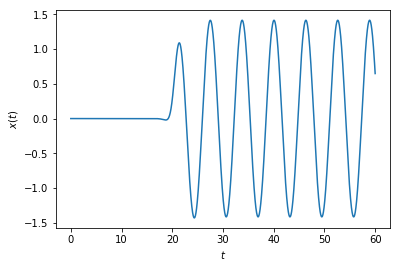In :
# 2(b) Ratio test
h0 = 0.01
t0s, x0s = twostep(h=4*h0)
t1s, x1s = twostep(h=2*h0)
t2s, x2s = twostep(h=h0)
ratios = (x0s[1::1] - x1s[1::2])/(x1s[1::2] - x2s[1::4])
plt.xlabel("$t$")
plt.ylabel("ratio")
plt.xlim(20, 60)# only show once the function has increased from zero
plt.ylim(0,6)
plt.plot(t0s[1:], ratios);

/Users/samdolan/anaconda/lib/python3.6/site-packages/ipykernel_launcher.py:6: RuntimeWarning: invalid value encountered in true_divide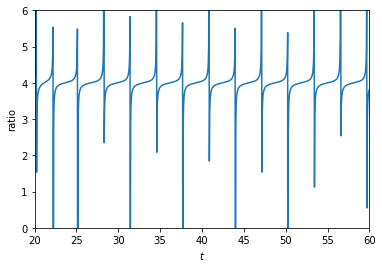In [ ]: